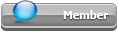## Featured Articles

Check out the latest featured articles.## File Library2# Real Gases Enthalpy As A Function Of Pressure

5 replies to this topic
|

### #1Qasem215

Qasem215

Junior Member

•• Members
•• 15 posts

Posted 21 January 2020 - 10:09 AM

Hello everyone, I'm a fresh grad but I still want to learn more and I've spent a lot of time probably looking in wrong places to find the change of enthalpy of a real gas as a function of pressure only. Could anyone guide me in the right direction?
My purpose is to understand how programs such as ASPEN calculate the thermodynamic properties, i would like to develop my own excel sheets and share them after learning how to do this.

### #2MrShorty

MrShorty

Gold Member

•• ChE Plus Subscriber
•• 471 posts

Posted 21 January 2020 - 11:45 AM   Best Answer

A few concepts that are important to this endeavor.

1) Even if you find derivations all done, understanding where many of these equations comes from begins by recalling the section of your physical chemistry and/or intro to thermodynamics courses that covered Maxwell's relations and how to use various properties of partial derivatives to build the partial differential equations at the heart of of most thermodynamic calculations. If you have kept your P-chem and/or thermo texts and notes, go back and review the sections dealing with Maxwell's relations (wikipedia's page: https://en.wikipedia...xwell_relations).

2) We often find it easier to measure and compute how far a property "departs" from an easily computed "ideal" or "standard" state. We then use these departure functions to compute the desired property (Wikipedia's page for departure functions -- including some equations fro the Peng-Robinson equation of state: https://en.wikipedia...arture_function).

That should give you a good start.

### #3breizh

breizh

Gold Member

•• ChE Plus Subscriber
•• 4,636 posts

Posted 21 January 2020 - 11:28 PM

Hi,

You may find some interest reading  the document I prepared a few years ago .

Hope this is helping you.

Good luck

Breizh

### #4Qasem215

Qasem215

Junior Member

•• Members
•• 15 posts

Posted 22 January 2020 - 07:30 AM

A few concepts that are important to this endeavor.

1) Even if you find derivations all done, understanding where many of these equations comes from begins by recalling the section of your physical chemistry and/or intro to thermodynamics courses that covered Maxwell's relations and how to use various properties of partial derivatives to build the partial differential equations at the heart of of most thermodynamic calculations. If you have kept your P-chem and/or thermo texts and notes, go back and review the sections dealing with Maxwell's relations (wikipedia's page: https://en.wikipedia...xwell_relations).

2) We often find it easier to measure and compute how far a property "departs" from an easily computed "ideal" or "standard" state. We then use these departure functions to compute the desired property (Wikipedia's page for departure functions -- including some equations fro the Peng-Robinson equation of state: https://en.wikipedia...arture_function).

That should give you a good start.

Thank you so much, I've used the equation and got results with about +90% accuracy compared to steam tables. Now I'll try derive it from first principal to full understand it.

Best wishes

Edited by Qasem215, 22 January 2020 - 07:31 AM.

### #5Qasem215

Qasem215

Junior Member

•• Members
•• 15 posts

Posted 22 January 2020 - 07:32 AM

Hi,
You may find some interest reading the document I prepared a few years ago .

Hope this is helping you.
Good luck
Breizh

Best

### #6Qasem215

Qasem215

Junior Member

•• Members
•• 15 posts

Posted 12 June 2020 - 07:07 AM

A few concepts that are important to this endeavor.

1) Even if you find derivations all done, understanding where many of these equations comes from begins by recalling the section of your physical chemistry and/or intro to thermodynamics courses that covered Maxwell's relations and how to use various properties of partial derivatives to build the partial differential equations at the heart of of most thermodynamic calculations. If you have kept your P-chem and/or thermo texts and notes, go back and review the sections dealing with Maxwell's relations (wikipedia's page: https://en.wikipedia...xwell_relations).

2) We often find it easier to measure and compute how far a property "departs" from an easily computed "ideal" or "standard" state. We then use these departure functions to compute the desired property (Wikipedia's page for departure functions -- including some equations fro the Peng-Robinson equation of state: https://en.wikipedia...arture_function).

That should give you a good start.

Thank you so much, I've used the equation and got results with about +90% accuracy compared to steam tables. Now I'll try derive it from first principal to full understand it.

Best wishes

I've created a spreadsheet based on the P-R EOS and I would like to add the data in the file you've attached, briezh, if you wouldn't mind and i'll upload it here (after making it look a bit more neat) for everyone's knowledge.

### Similar Topics